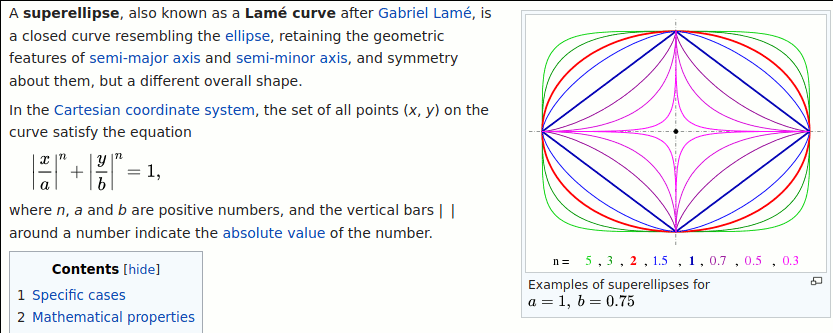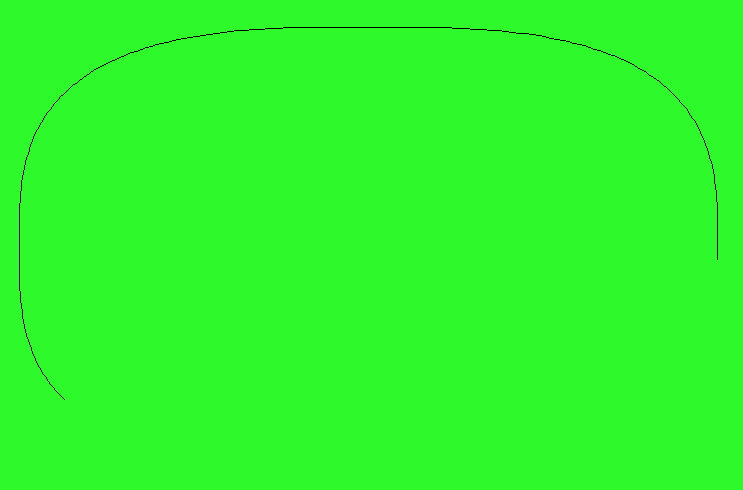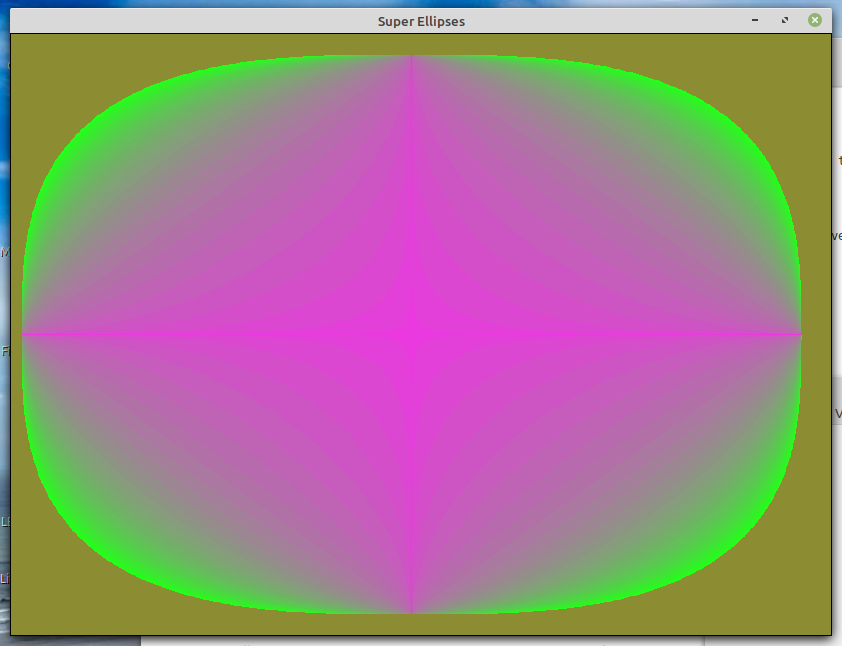# Super-ellipses

As Wikipedia puts it....These are a fun generalisation of ellipses. Piet Hein popularised the 3-D version decades ago as they form convincing 'egg' shapes which happily balance on their 'long' end!-It is fun to investgate by drawing a 'family' of super-ellipses. Then I added colour filling, saved each frame, and made this animation..You may prefer to set the boundary to fill TO to the same as the one to fill WITH.## Code

```    nomainwin

WindowWidth = 830  : WindowHeight = 630
UpperLeftX  =  10  : UpperLeftY   =  10

pi  =atn( 1) *4
Cx  =400
Cy  =300

open "Super Ellipses" For graphics_nsb as #wg

hw      =hwnd( #wg)
calldll #user32, "GetDC", hw as ulong, hdc as ulong

#wg "down; trapclose [quit]"
#wg "fill 140 140 50"

a   =390  '   horizontal semi-diameter
b   =280  '   vertical   semi-diameter
'n   =         exponent

r  = 30 '   Initial colour values for the fill.
g  =260
bl = 20

count =0

for n =3 to 0.1 step -0.1
#wg "down"

r           =r  +7       'int( 40 +215 *rnd( 1))                '   to create a colour gradient.
g           =g  -7       'int( 40 +215 *rnd( 1))                '   randoms f you prefer them..
bl          =bl +7       'int( 40 +216 *rnd( 1))

fillCol\$    =str\$( r)     +" " +str\$( g)   +" "   +str\$( bl)

#wg "color ";     fillCol\$
#wg "backcolor "; fillCol\$

targetcolor   =bl *2^16    +g *2^8    +r      '   this is colour to fill WITH
'   this is also the colour of the outline to fill out TO.

#wg "up ; goto "; 400 +a; " 300 ; down"

for t =0 to 2 *pi +0.02 step 0.01
'         oscillating term         semiaxes    switch quadrants term
x       =abs( cos( t))^( 2 /n)       *a          *sgn( cos( t))
y       =abs( sin( t))^( 2 /n)       *b          *sgn( sin( t))
xS      =400 +int( x)
yS      =300 +int( y)
#wg "goto "; xS; " "; yS
scan
'timer 50, [go]
'    wait
[go]
'timer 0
next t

calldll #gdi32, "ExtFloodFill",_
hdc                as ulong,_
Cx                 as  long,_
Cy                 as  long,_
targetcolor        as  long,_                '   ie fill out 'til this colour is met..
_FLOODFILLBORDER   as  long,_
result             as  long

#wg "getbmp scr 0 0 800 630"
'bmpsave "scr", "images/sc" +right\$( "000" +str\$( count), 3) +".bmp"
count =count +1
next n

wait

function sgn( w)
select case
case w <0
sgn =-1
case w =0
sgn = 0
case w >0
sgn = 1
end select
end function

[quit]
calldll #user32, "ReleaseDC", hw as ulong, hdc as ulong, ret as void   'release the DC
close #wg
end

```